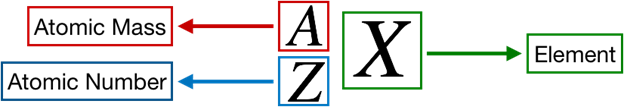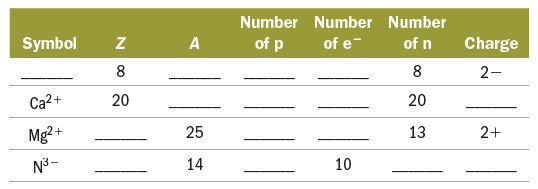Problem: Complete the following table:

FREE Expert Solution
84% (118 ratings)
FREE Expert Solution

We’re being asked to complete the fourth column of the given table.

Recall that the symbol for an element or ion is as follows:Recall that the number of protons is equal to the atomic number.

For the first row:

The atomic number Z = 8 is already givenThis means that:

84% (118 ratings)
Problem Details

Complete the following table:What scientific concept do you need to know in order to solve this problem?

Our tutors have indicated that to solve this problem you will need to apply the Ions concept. You can view video lessons to learn Ions Or if you need more Ions practice, you can also practice Ions practice problems .

How long does this problem take to solve?

Our expert Chemistry tutor, Jules took 3 minutes to solve this problem. You can follow their steps in the video explanation above.

What professor is this problem relevant for?

Based on our data, we think this problem is relevant for Professor Hillyard's class at CSU CHICO.

What textbook is this problem found in?

Our data indicates that this problem or a close variation was asked in . You can also practice practice problems .